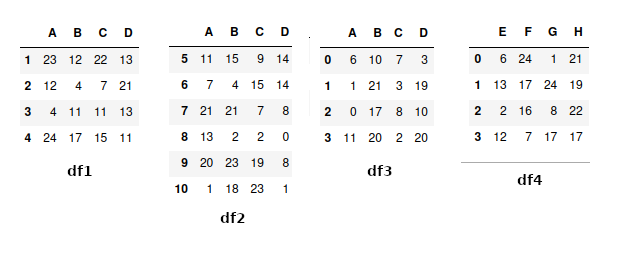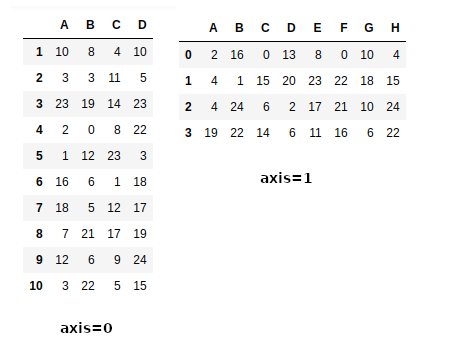GeeksforGeeks App
Open AppBrowser
Continue

# How To Concatenate Two or More Pandas DataFrames?

Let’s understand how we can concatenate two or more Data Frames. A concatenation of two or more data frames can be done using pandas.concat() method. concat() in pandas works by combining Data Frames across rows or columns. We can concat two or more data frames either along rows  (axis=0) or along columns (axis=1)

## Creating Dataframe to Concatenate Two or More Pandas DataFrames

Create two Data Frames which we will be concatenating now. For creating Data frames we will be using numpy and pandas.

## Python3

 `import` `pandas as pd``import` `numpy as np` `df1 ``=` `pd.DataFrame(np.random.randint(``25``, size``=``(``4``, ``4``)),``                   ``index``=``[``"1"``, ``"2"``, ``"3"``, ``"4"``],``                   ``columns``=``[``"A"``, ``"B"``, ``"C"``, ``"D"``])` `df2 ``=` `pd.DataFrame(np.random.randint(``25``, size``=``(``6``, ``4``)),``                   ``index``=``[``"5"``, ``"6"``, ``"7"``, ``"8"``, ``"9"``, ``"10"``],``                   ``columns``=``[``"A"``, ``"B"``, ``"C"``, ``"D"``])` `df3 ``=` `pd.DataFrame(np.random.randint(``25``, size``=``(``4``, ``4``)),``                   ``columns``=``[``"A"``, ``"B"``, ``"C"``, ``"D"``])` `df4 ``=` `pd.DataFrame(np.random.randint(``25``, size``=``(``4``, ``4``)),``                   ``columns``=``[``"E"``, ``"F"``, ``"G"``, ``"H"``])` `display(df1, df2, df3, df4)`

Output:DataFrame with some random data for testing

## Concatenate Two or More Pandas DataFrames

We’ll pass two dataframes to pd.concat() method in the form of a list and mention in which axis you want to concat, i.e. axis=0 to concat along rows, axis=1 to concat along columns.

## Python3

 `# concatenating df1 and df2 along rows``vertical_concat ``=` `pd.concat([df1, df2], axis``=``0``)` `# concatenating df3 and df4 along columns``horizontal_concat ``=` `pd.concat([df3, df4], axis``=``1``)` `display(vertical_concat, horizontal_concat)`

Output:Output DataFrame for different axis parameter values

My Personal Notes arrow_drop_up